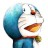# 杰拉斯的博客杰拉斯 | 时间：2012-04-18, Wed | 21,649 views

Time Limit: 2000/1000 MS (Java/Others) Memory Limit: 65536/32768 K (Java/Others)
Total Submission(s): 22404 Accepted Submission(s): 6972

#### Description

FatMouse prepared M pounds of cat food, ready to trade with the cats guarding the warehouse containing his favorite food, JavaBean.
The warehouse has N rooms. The i-th room contains J[i] pounds of JavaBeans and requires F[i] pounds of cat food. FatMouse does not have to trade for all the JavaBeans in the room, instead, he may get J[i]* a% pounds of JavaBeans if he pays F[i]* a% pounds of cat food. Here a is a real number. Now he is assigning this homework to you: tell him the maximum amount of JavaBeans he can obtain.

#### Input

The input consists of multiple test cases. Each test case begins with a line containing two non-negative integers M and N. Then N lines follow, each contains two non-negative integers J[i] and F[i] respectively. The last test case is followed by two -1's. All integers are not greater than 1000.

#### Output

For each test case, print in a single line a real number accurate up to 3 decimal places, which is the maximum amount of JavaBeans that FatMouse can obtain.

```
5 3
7 2
4 3
5 2
20 3
25 18
24 15
15 10
-1 -1
```

```
13.333
31.500
```

#### Source

HDU1009

```#include<stdio.h>
#include<vector>
#include<algorithm>
using namespace std;
struct room{
int j, f;
bool operator < (const room t) const{
return (float)j / f > (float)t.j / t.f;
}

};
int main(){
int m, n, i;
while(scanf("%d%d", &m, &n) && (m + 1) || (n + 1)){
vector<room> v;
room r;
double ans = 0;
for(i = 0; i < n; ++i){
scanf("%d%d", &r.j, &r.f);
v.push_back(r);
}
sort(v.begin(), v.end());
i = 0;
while(m > 0 && i < v.size()){
if(v[i].f <= m){
m -= v[i].f;
ans += v[i].j;
}else{
ans += (double)m / v[i].f * v[i].j;
m = 0;
}
++i;
}
printf("%.3lf\n", ans);
}
return 0;
}
```